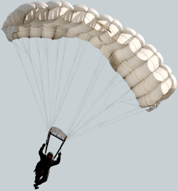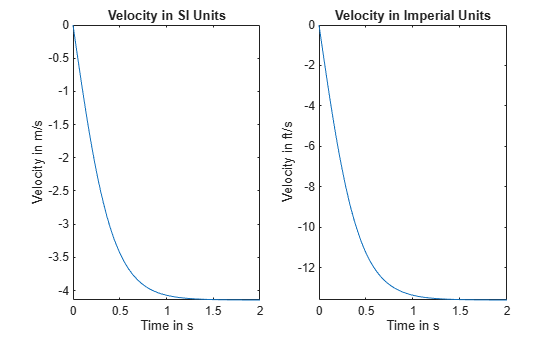# Units in Physics Calculations

This example shows how to work with units in physics calculations. Calculate the terminal velocity of a falling paratrooper in both SI and imperial units. Solve the motion of the paratrooper, taking into account the gravitational force and the drag force.

### Introduction

Imagine a paratrooper jumping out of an airplane. Assume there are only two forces acting on the paratrooper: the gravitational force and an opposing drag force from the parachute. The drag force is proportional to the velocity squared of the paratrooper.The net force acting on the paratrooper can be expressed as

$\mathrm{mass}\cdot \mathrm{acceleration}=\mathrm{drag}\text{\hspace{0.17em}}\mathrm{force}-\mathrm{gravitational}\text{\hspace{0.17em}}\mathrm{force}$,

$\mathit{m}\frac{\partial }{\partial \mathit{t}}\mathit{v}\left(\mathit{t}\right)={\mathit{c}}_{\mathit{d}}{\mathit{v}\left(\mathit{t}\right)}^{2}-\mathit{m}\text{\hspace{0.17em}}\mathit{g}$,

where

• $m$ is the mass of the paratrooper

• g is the gravitational acceleration

• $v\left(t\right)$is the velocity of the paratrooper

• ${c}_{d}$ is the drag constant

### Define and Solve Equation of Motion

Define the differential equation describing the equation of motion.

```syms g m c_d syms v(t) eq = m*diff(v(t),t) + m*g == c_d*v(t)^2```
```eq =  ```

Assume that the parachute opens immediately at $\mathit{t}=0$ so that the equation `eq` is valid for all values of $\mathit{t}\ge 0$. Solve the differential equation analytically using `dsolve` with the initial condition $\mathit{v}\left(0\right)=0$. The solution represents the velocity of the paratrooper as a function of time.

`velocity = simplify(dsolve(eq, v(0) == 0))`
```velocity =  $-\frac{\sqrt{g} \sqrt{m} \mathrm{tanh}\left(\frac{\sqrt{{c}_{d}} \sqrt{g} t}{\sqrt{m}}\right)}{\sqrt{{c}_{d}}}$```

### Find Unit of Drag Constant

Find the SI unit of the drag constant ${c}_{d}$.

The SI unit of force is the Newton $\left(\mathit{N}\right)$. In terms of the base units, the Newton is $\left(\frac{\mathrm{kg}\cdot \mathit{m}}{{\mathit{s}}^{2}}\right)$. Since these are equivalent, they have a unit conversion factor of 1.

```u = symunit; unitConversionFactor(u.N, u.kg*u.m/u.s^2)```
`ans = $1$`

The drag force ${\mathit{c}}_{\mathit{d}}{\mathit{v}\left(\mathit{t}\right)}^{2}$ must have the same unit in Newton $\left(\mathit{N}\right)$ as the gravitational force $m\phantom{\rule{0.16666666666666666em}{0ex}}g$. Using dimensional analysis, solve for the unit of ${c}_{d}$.

```syms drag_units_SI drag_units_SI = simplify(solve(drag_units_SI * (u.m / u.s)^2 == u.N))```
```drag_units_SI =  $\frac{\mathrm{kg}\mathrm{"kilogram - a physical unit of mass."}}{\mathrm{m}\mathrm{"meter - a physical unit of length."}}$```

### Estimate Terminal Velocity

Describe the motion of the paratrooper by defining the following values.

• Mass of the paratrooper $\mathit{m}\text{\hspace{0.17em}}=\text{\hspace{0.17em}}70\text{\hspace{0.17em}}\mathrm{kg}$

• Gravitational acceleration $\mathit{g}\text{\hspace{0.17em}}=\text{\hspace{0.17em}}9.81\text{\hspace{0.17em}}\mathit{m}/{\mathit{s}}^{2}$

• Drag coefficient ${\mathit{c}}_{\mathit{d}}\text{\hspace{0.17em}}=\text{\hspace{0.17em}}40\text{\hspace{0.17em}}\mathrm{kg}/\mathit{m}$

Substitute these values into the velocity equation and simplify the result.

`vel_SI = subs(velocity,[g,m,c_d],[9.81*u.m/u.s^2, 70*u.kg, 40*drag_units_SI])`
```vel_SI =  $-\frac{\mathrm{tanh}\left(\frac{t \sqrt{40 \frac{\mathrm{kg}\mathrm{"kilogram - a physical unit of mass."}}{\mathrm{m}\mathrm{"meter - a physical unit of length."}}} \sqrt{\frac{981}{100} \frac{\mathrm{m}\mathrm{"meter - a physical unit of length."}}{{\mathrm{s}\mathrm{"second - a physical unit of time."}}^{2}}}}{\sqrt{70 \mathrm{kg}\mathrm{"kilogram - a physical unit of mass."}}}\right) \sqrt{70 \mathrm{kg}\mathrm{"kilogram - a physical unit of mass."}} \sqrt{\frac{981}{100} \frac{\mathrm{m}\mathrm{"meter - a physical unit of length."}}{{\mathrm{s}\mathrm{"second - a physical unit of time."}}^{2}}}}{\sqrt{40 \frac{\mathrm{kg}\mathrm{"kilogram - a physical unit of mass."}}{\mathrm{m}\mathrm{"meter - a physical unit of length."}}}}$```
`vel_SI = simplify(vel_SI)`
```vel_SI =  $-\frac{3 \sqrt{763} \mathrm{tanh}\left(\frac{3 \sqrt{763} t}{35} \frac{1}{\mathrm{s}\mathrm{"second - a physical unit of time."}}\right)}{20} \frac{\mathrm{m}\mathrm{"meter - a physical unit of length."}}{\mathrm{s}\mathrm{"second - a physical unit of time."}}$```

Compute a numerical approximation of the velocity to 3 significant digits.

```digits(3) vel_SI = vpa(vel_SI)```
```vel_SI =  $-4.14 \mathrm{tanh}\left(2.37 t \frac{1}{\mathrm{s}\mathrm{"second - a physical unit of time."}}\right) \frac{\mathrm{m}\mathrm{"meter - a physical unit of length."}}{\mathrm{s}\mathrm{"second - a physical unit of time."}}$```

The paratrooper approaches a constant velocity when the gravitational force is balanced by the drag force. This is called the terminal velocity and it occurs when the drag force from the parachute cancels out the gravitational force (there is no further acceleration). Find the terminal velocity by taking the limit of $\mathit{t}⟶\infty$.

`vel_term_SI = limit(vel_SI, t, Inf)`
```vel_term_SI =  $-4.14 \frac{\mathrm{m}\mathrm{"meter - a physical unit of length."}}{\mathrm{s}\mathrm{"second - a physical unit of time."}}$```

### Convert Velocity to Imperial Units

Finally, convert the velocity function from SI units to imperial units.

`vel_Imperial = rewrite(vel_SI,u.ft)`
```vel_Imperial =  $-13.6 \mathrm{tanh}\left(2.37 t \frac{1}{\mathrm{s}\mathrm{"second - a physical unit of time."}}\right) \frac{\mathrm{ft}\mathrm{"foot - a physical unit of length."}}{\mathrm{s}\mathrm{"second - a physical unit of time."}}$```

Convert the terminal velocity.

`vel_term_Imperial = rewrite(vel_term_SI,u.ft)`
```vel_term_Imperial =  $-13.6 \frac{\mathrm{ft}\mathrm{"foot - a physical unit of length."}}{\mathrm{s}\mathrm{"second - a physical unit of time."}}$```

### Plot Velocity over Time

To plot the velocity as a function of time, express the time `t` in seconds and replace `t` by `T` s, where `T` is a dimensionless symbolic variable.

```syms T vel_SI = subs(vel_SI, t, T*u.s)```
```vel_SI =  $-4.14 \mathrm{tanh}\left(2.37 T\right) \frac{\mathrm{m}\mathrm{"meter - a physical unit of length."}}{\mathrm{s}\mathrm{"second - a physical unit of time."}}$```
`vel_Imperial = rewrite(vel_SI, u.ft)`
```vel_Imperial =  $-13.6 \mathrm{tanh}\left(2.37 T\right) \frac{\mathrm{ft}\mathrm{"foot - a physical unit of length."}}{\mathrm{s}\mathrm{"second - a physical unit of time."}}$```

Separate the expression from the units by using `separateUnits`. Plot the expression using `fplot`. Convert the units to strings for use as plot labels by using `symunit2str`.

```[data_SI, units_SI] = separateUnits(vel_SI); [data_Imperial, units_Imperial] = separateUnits(vel_Imperial);```

The velocity of the paratrooper approaches steady state when $\mathit{t}>1$. Show how the velocity approaches terminal velocity by plotting the velocity over the range $0\text{\hspace{0.17em}}\le \mathit{T}\text{\hspace{0.17em}}\le 2$.

```subplot(1,2,1) fplot(data_SI,[0 2]) title('Velocity in SI Units') xlabel('Time in s') ylabel(['Velocity in ' symunit2str(units_SI)]) subplot(1,2,2) fplot(data_Imperial,[0 2]) title('Velocity in Imperial Units') xlabel('Time in s') ylabel(['Velocity in ' symunit2str(units_Imperial)])```